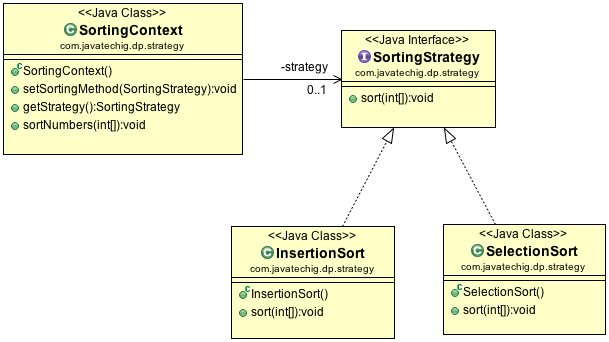The Strategy design pattern belongs to the behavioral family of pattern that deals with the change of the behavior of a class by changing the internal algorithm at runtime without modifying the class itself. This allows extensibility and loose coupling of objects. The definition of State Design Pattern as per the  original Gang of Four book is;

“Defines a set of encapsulated algorithms that can be swapped to carry out a specific behavior.”

### Use cases

A data compression software like WinZip, provides different algorithms to perform gip, gzip, tar, jar, 7zip format. At runtime, the client chooses which type of algorithm to be performed.

Email client like outlook supports various email types such as plain text and HTML type. It allows the client to choose the email format.

### How does Strategy Pattern Works?

The Strategy pattern is simple yet popular design pattern mostly works together with State pattern. Following are the steps involved to create strategy design pattern:

1. Implement a Strategy interface for your strategy objects. This interface defines the strategy object behavior.
2. Implement Concrete Strategy classes that implement the Strategy interface defined in the above step.
3. Create a Context class and maintain the reference to strategy object. Create a setter and getter method to allow access to a strategy object.

### Strategy Design Pattern Example

Let us take the example of a program that performs various number sorting algorithm such as Insertion sort, Selection Sort, Merge Sort etc. It allows the client to choose which type of sorting he would like to perform.

The above use case can be represented in the following class diagramSortingStrategy.java

```public interface SortingStrategy {

public void sort(int[] numbers);

}```

SelectionSort.java

```public class SelectionSort implements SortingStrategy {

@Override
public void sort(int[] numbers) {
System.out.println("Selection Sort!");

int i, j, first, temp;
for (i = numbers.length - 1; i > 0; i--) {
first = 0;
for (j = 1; j <= i; j++) {
if (numbers[j] > numbers[first])
first = j;
}
temp = numbers[first];
numbers[first] = numbers[i];
numbers[i] = temp;
}

System.out.println(Arrays.toString(numbers));
}
}
```

InsertionSort.java

```public class InsertionSort implements SortingStrategy {

@Override
public void sort(int[] numbers) {
System.out.println("Insertion Sort!");

for (int i = 1; i < numbers.length; i++) {
int temp = numbers[i];
int j;
for (j = i - 1; (j >= 0) && (numbers[j] > temp); j--) {
numbers[j + 1] = numbers[j];
}
numbers[j + 1] = temp;
}

System.out.println(Arrays.toString(numbers));
}
}
```
```public class SortingContext {

private SortingStrategy strategy;

public void setSortingMethod(SortingStrategy strategy) {
this.strategy = strategy;
}

public SortingStrategy getStrategy() {
return strategy;
}

public void sortNumbers(int[] numbers){
strategy.sort(numbers);
}
}
```

TestMain.java

Here is how client using strategy pattern

```public class TestMain {

public static void main(String[] args) {

int numbers[] = {20, 50, 15, 6, 80};

SortingContext context = new SortingContext();
context.setSortingMethod(new InsertionSort());
context.sortNumbers(numbers);

System.out.println("***********");
context.setSortingMethod(new SelectionSort());
context.sortNumbers(numbers);

}
}
```

Output

```Insertion Sort!
[6, 15, 20, 50, 80]
***********
Selection Sort!
[6, 15, 20, 50, 80]

```